# Nyquist Plot for Impedance Measurement of Lithium-ion Batteries

## What is the Nyquist plot (Cole-Cole plot)?

A Nyquist plot is a graph that plots impedance values measured at multiple frequencies. Impedance is a parameter that contains the resistance and reactance components.

•Fig. 1: Typical Nyquist plot of internal resistance of a Lithium-ion battery

## What is the impedance measurement method?

It is a method of measuring impedance by applying an AC signal. The AC signal used to measure the impedance of a battery usually has a fixed frequency of 1 kHz. There is also a method for measuring impedance using several frequencies as opposed to a single frequency. It is called Electrochemical Impedance Spectroscopy, or EIS. This method does not require disassembling the battery so it provides a safe and non-destructive way to observe the behavior inside a battery.

The internal resistance of the battery in general can be broadly divided into the following three categories.

1. Electrolyte resistance
2. Reaction resistance
3. Diffusion resistance

As shown in Fig.  2, the physical phenomena that dominate impedance differ depending on the frequency band. For example, the impedance at high frequencies (about 1 kHz) is mostly caused by Lithium-ion migration in the electrolyte. Lithium-ion diffusion occurs within the electrode at low frequencies (less than 1 Hz) and Li-ion transfer reactions at intermediate frequencies (1 to several hundreds of Hz). In other words, a detailed analysis of the Nyquist plot allows us to evaluate various phenomena in different parts of the battery.

•Fig. 2: Typical relation between Nyquist plot and internal resistance of a battery

A typical analysis method is known as equivalent circuit analysis, which uses an equivalent circuit model as shown in Fig. 3. In the circuit model, each phenomenon inside the battery is modeled by different equivalent circuit elements. The element values calculated by the analysis can be considered to indicate the characteristics of the physical phenomena represented by the elements.

•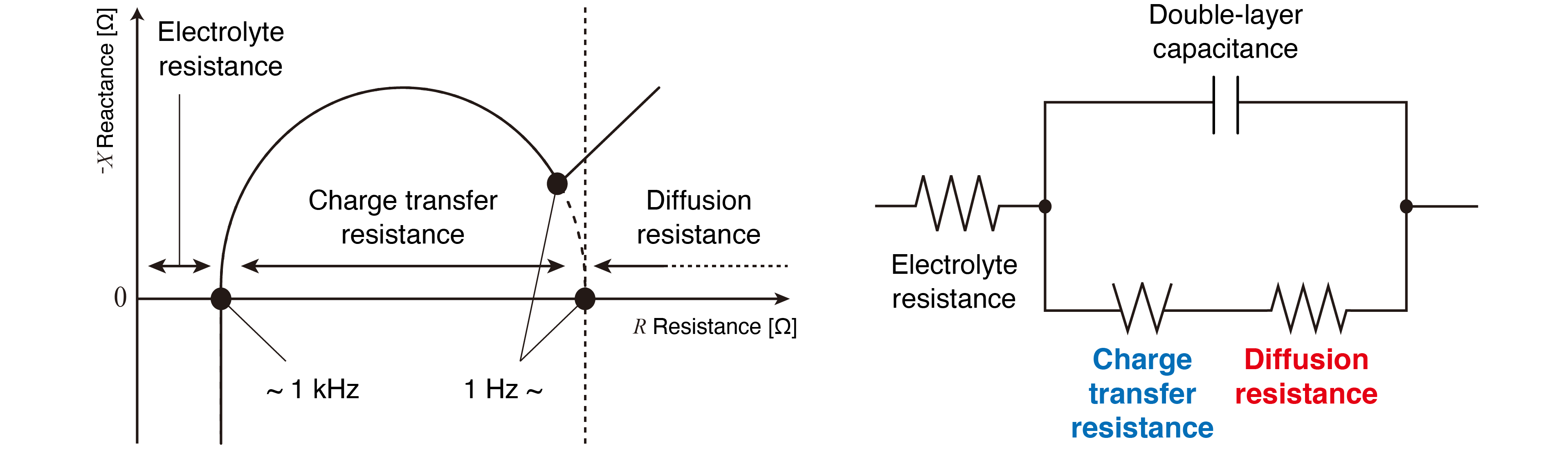Fig. 3: Example of Nyquist Plot and Equivalent Circuit Model

## Applications

### 1. Checking the battery deterioration level

As shown in Fig. 4, there is a significant difference in the reaction resistance between a pristine and a degraded Lithium-ion battery. In applications that involve charging and discharging at low temperatures or deep charging and discharging (SOC between 0% and 100%), degradation is likely to occur. The degradation of charge transfer resistance is particularly noticeable in the Nyquist plot as reaction resistance.

•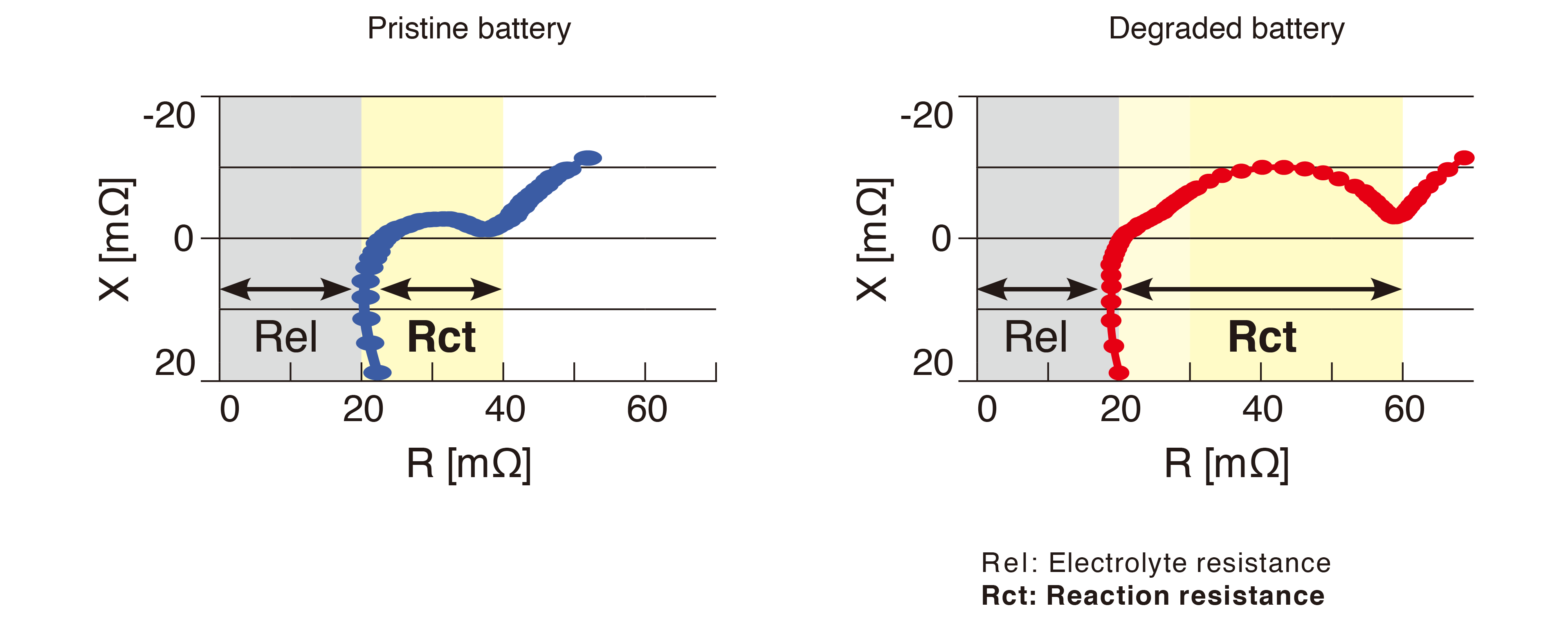Fig. 4: Comparing the measured data for pristine and deteriorated lithium-ion batteries with the Nyquist plot

### 2. Identify battery deterioration factors

The causes of battery cell defects can be identified by measuring AC impedance at multiple frequencies. For example,

•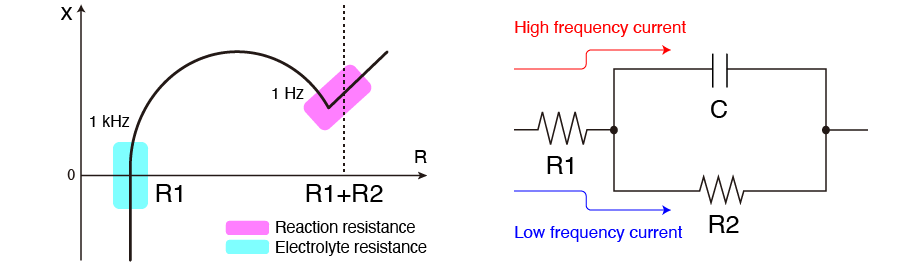When R1 is larger: Decreasing in electrolyte concentration, or the electrode might have poor welding.
When R2 is larger: A failure might have occurred during the electrode production process, or the electrode might react poorly on its surface.

## Solutions proposed by Hioki

### 1. Measuring instruments

Product model Purpose Appropriate battery type BT4560 IM3590 IM3536-01+9268-10 Cell production line, For R&D (Research and Development) For R&D (Research and Development) For R&D (Research and Development) Lithium-ion battery All-solid-state battery All-solid-state battery 5 V max. 5 V max. 10 V max. 0.1 Hz to 1.05 kHz *0.04 Hz to 10 kHz 1 mHz to 200 kHz 4 Hz to 10 MHz
• *Special specification

### 2. Multiple measurement and Evaluation

The SW1001 and SW1002 are switch mainframes for multiplexer modules that let you test batteries quickly and on more than one channel. Use one of them in combination with the BT4560 or the IM3590 to perform multi-channel Nyquist plot measurements.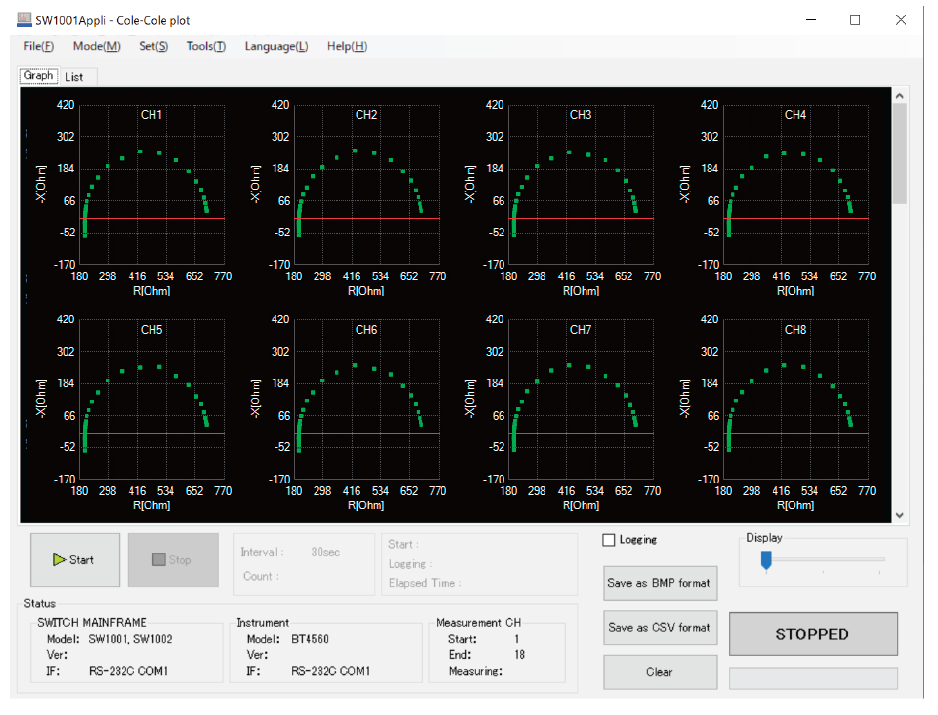### 3. Multi-plot

"Multi-plot", a free web application, enables you to draw a Nyquist plot simply by loading a file in your web browser. Freely draw the Nyquist plot without any limits on the number of points that can be drawn from a file or the number of graphs that can be overlapped. The horizontal and vertical axes are automatically scaled based on the graphs being rendered. Files acquired with different measuring instruments can also be overlapped for comparison and analysis.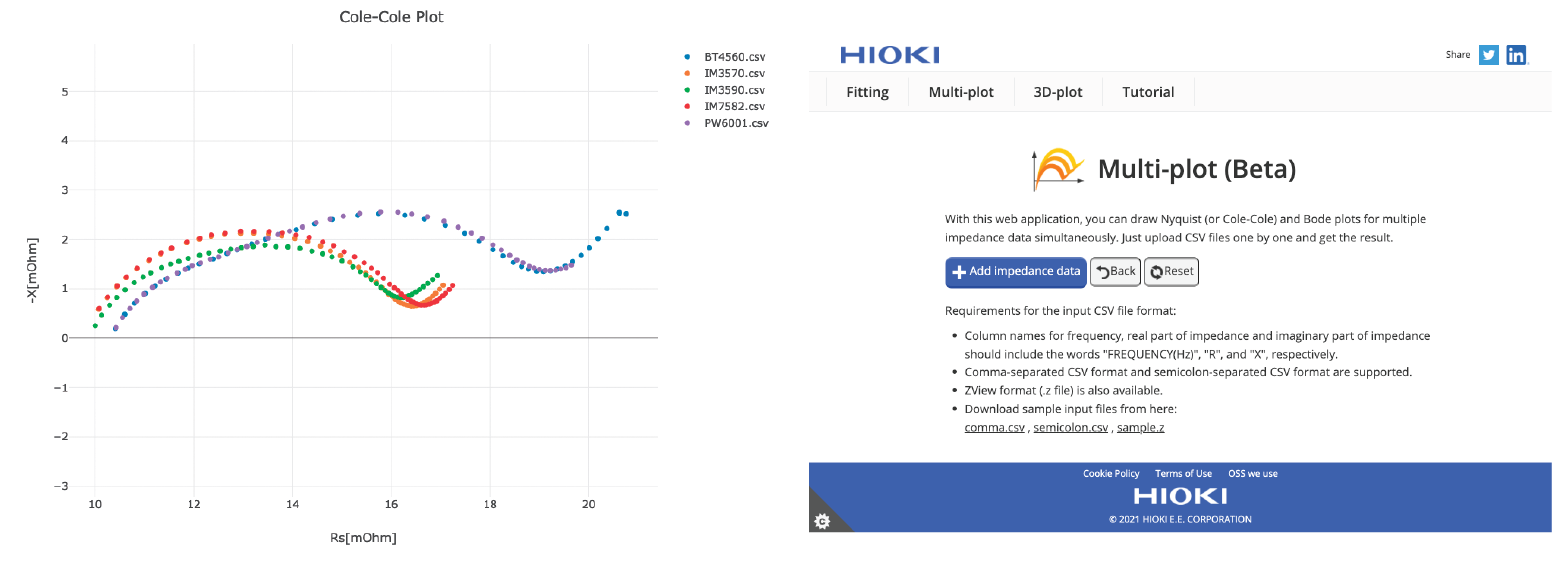Sustainable society cannot be realized without the development and production of high-quality batteries. We at Hioki offer a wide range of battery measurement solutions.